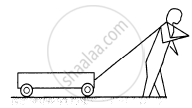Department of Pre-University Education, KarnatakaPUC Karnataka Science Class 11

# Figure Shows a Boy Pulling a Wagon on a Road. List as Many Forces as You Can Which Are Relevant with this Figure. - Physics

Short Note

Figure shows a boy pulling a wagon on a road. List as many forces as you can which are relevant with this figure. Find the pairs of forces connected by Newton's third law of motion.#### Solution

List of forces :

(a) A pair of gravitational force between the wagon and the Earth.
(b) A frictional force exerted by the road on the wagon.
(c) A tension exerts electromagnetic force between the wagon and string.
(d) A pair of gravitational force between the man and the Earth.
(e) A frictional force exerted by the road on the man.
(f) A tension exerts electromagnetic force between the man and string.
Here, (a), (c), (d) and (f) are pairs of forces associated with Newton's third law of motion.

Concept: Work Done by a Constant Force and a Variable Force
Is there an error in this question or solution?

#### APPEARS IN

HC Verma Class 11, Class 12 Concepts of Physics Vol. 1
Chapter 4 The Forces
Short Answers | Q 9 | Page 61
Share